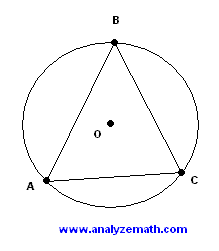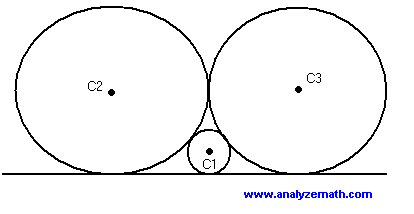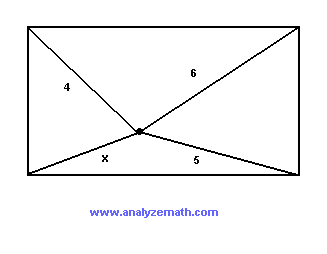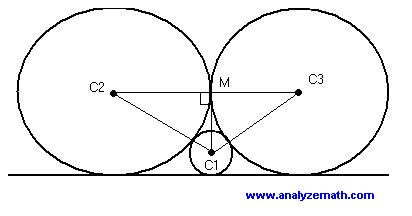Grade 12 geometry problems with detailed solutions are presented. These geometry problems are presented here to help you think and learn how to solve problems. Do not give up quickly if a problem is a challenging one. Spend time solving these problems and work in groups if possible as group work encourages you to discuss ideas and learn from each other. We learn by solving problems that we do not know how solve at first.

Problems

1. In the triangle ABC sides AB and CB have equal lengths and the measure of angle ABC is equal to 36 degrees. What is the measure of angle BOC where O is the center of the circle?.

2. Circles C1 and C2 have equal radii and are tangent to that same line L. Circle C3 is tangent to C1 and C2. x is the distance between the between the centers of C1 and C2. Find the distance h, from the center of C3 to line L, in terms of x and the radii of the three circles..

3. All three circles are tangent to the same line and to each other. Circles C2 and C3 have equal radii. Find the radius of C2 if the radius of C1 is equal to 10 cm..

4. CD is parallel to AB and the measure of angle t is equal to 90 degrees. Find the area of the circle in terms of x..

5. The shaded region below is the common area to four semicircles whose diameters are the sides of the square with side length 4x. Find the area of the shaded region in terms of x..

6. The two circles below are concentric (have same center). The length of the chord tangent to the smaller circle is equal to 20 mm. What is the area of the ring (shaded area) between the two circles?.

7. Find a, b and c so that the quadrilateral is a parallelogram with area equal to 80 square units..

8. A right triangle is shown below. Find the lengths x, y and z..

9. A rectangle is shown below. Find the length x..

10. The two circles below have equal radii of 4 units each and the distance between their centers is 6 units. Find the area of the shaded region..

Solutions to the Above Problems

1. measure of angle BAC = (180 - 36)/2 = 72 degrees : isosceles triangle
measure of angle BOC = 2 * measure of angle BAC = 144 degrees : inscribed angle and central angle intercepting the same arc.

2. Let R1, R2 and R3 be the radii of circles C1, C2 and C3 respectively with R1 = R2 = R
h = C3O + R
C3O2 + (x/2)2 = (R + R3)2 : Pythagora's theorem applied to triangle C3OC1.
h = R + C3O = R + √[ (R + R3)2 - (x/2)2 ].

3. Let r, R2 and R3 be the radii of circles C1, C2 and C3 respectively with R2 = R3 = R
(r + R)2 = R2 + (R - r)2 : Pythagora's theorem applied to triangle MC1C3
R = 4r = 40 cm : expand and solve for R..

4. The measure of angle BtA is equal to 90 degrees : vertical angles
AB is the diameter of the circle : converse of Thales theorem
triangles BtA and CtD are similar : CD parallel to AB
3/5 = AB/x : corresponding sides proportional.
AB = 3x/5 : solve for AB
area = Pi(3x/10)2 = 0.09 Pi x2

5. The square below has side length 2x, half of the given square. Part of this is shaded and the other part is not shaded. Let us find the area of the non shaded part (white). The shaded part is a quarter of a disk (circle).
area of non shaded area = (2x)2 - (1/4) Pi (2x)2
If we go back to the given shape in problem 5, the area of the non shaded part is 8 times the non shaded area in the present shape which was calculated above.
area of shaded part in shape of problem 5 = total area of square - total non shaded area = (4x)2 - 8*[ (2x)2 - (1/4) Pi (2x)2 ]
= 16x2(Pi/2 - 1).

6. R2 = r2 + 102 : Pythagora's theorem
Pi(R2 - r2) = 100 Pi : area of ring.

7. The area of a parallelogram may be calculated using the cross product of vectors AB and AD as follows.
area = |AB x AD| where AB and AD are 3-dimensional vectors.
vector AB = <4 , b + 2 , 0> : set third component to zero since the given shape is 2-dimensional.
vector AD = <6 , 4 , 0>
|<4 , b + 2 , 0> x <6 , 4 , 0>| = 80 : modulus of cross product is equal to area
|4 - 6b| = 80
b = 14 and b = -38/3 : we select the solution b = 14 since point B(2,b) is in quadrant I.
Since ABCD is a parallelogram, vector AB = vector DC
Vector AB = <4 , 16 , 0>
vector DC =
c - 4 = 4 and d - 2 = 16 : If two vectors are equal their corresponding components area equal.
c = 8 and d = 18 : solve the above equations.

8. y2 + z2 = 122 : Pythagora's theorem
x2 + z2 = 92 : Pythagora's theorem
(y + x)2 = 122 + 92 : Pythagora's theorem
x + y = 15 : Solve equation C by extracting the square root
y2 - x2 = 63 : subtracting equations A and B
(y - x)(y + x) = 63 : factoring the left term of equation E.
y - x = 21/5
x = 27/5 , y = 48/5 and z = 36/5 : solve the system made up of equations D and G.

9. Split the given rectangle into 4 other rectangles as shown.
a2 + c2 = 42 : Pythagora's theorem applied to top left right triangle.
b2 + c2 = x2 : Pythagora's theorem applied to bottom left right triangle.
b2 + d2 = 52 : Pythagora's theorem applied to bottom right right triangle.
a2 + d2 = 62 : Pythagora's theorem applied to bottom right right triangle.
a2 - b2 = 42 - x2 : subtract equations B and C.
a2 - b2 = 62 - 52 : subtract equations D and E.
42 - x2 = 62 - 52 : combine equations F and G.
x = √5 : solve above equation for x..

10. Because of symmetry, the shaded region may be considered as made up of two equal (in area) regions. The area of the left half of the shaded region is given by the area of the sector BOC minus the area of the triangle BOC.
length of OM = 3 (by symmetry) since distance between centers is 6, and radius r = 4.
Let t be the measure of angle BOM.
cos(t) = OM/OB = 3/4 , t = arccos(3/4) : using right triangle BOM.
area of sector BOC = (1/2)(2t)r2
area of triangle BOC = (1/2)sin(2t)r2
area of shaded region = 2 [ (1/2)(2t)r2 - (1/2)sin(2t)r2 ]
= [2t - sin(2t)] r2 = 7.25 square units (rounded to 3 decimal places).

More
High School Math (Grades 10, 11 and 12) - Free Questions and Problems With Answers
More
Middle School Math (Grades 6, 7, 8, 9) - Free Questions and Problems With Answers
More
Primary Math (Grades 4 and 5) with Free Questions and Problems With Answers